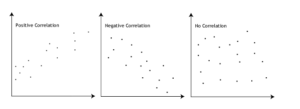# HOW TO RUN SPEARMAN'S RANK CORRELATION TEST IN SPSS

## What is Spearman's Correlation Test?

### Using Spearman’s Correlation Statistic in Research

This easy tutorial will show you how to run Spearman’s Correlation test in SPSS, and how to interpret the result.

The Spearman correlation coefficient is the non-parametric equivalent of the Pearson correlation coefficient. It similarly takes values between -1 and +1, but the difference is that it quantifies the extent to which the variables tend to increase or decrease together i.e., the extent to which one variable tends to increase as the other increases or decreases. A value of zero indicates no such tendency. (Source)

We can categorize the type of correlation by considering as one variable increases what happens to the other variable:

• Positive correlation – the other variable has a tendency to also increase;
• Negative correlation – the other variable has a tendency to decrease;
• No correlation – the other variable does not tend to either increase or decrease.

The starting point of any such analysis should be the construction and subsequent examination of a scatterplot. Examples of negative, no, and positive correlation are as follows.### Are there guidelines for interpreting Spearman’s correlation coefficient?

Yes, We proposed the following guidelines: A Spearman’s correlation coefficient between 0.51 and 0.99 indicates a high correlation between variables (values above 0.80 indicate an extremely high correlation. )

### Assumptions of the Spearman’s Correlation Test

The assumptions for Spearman’s correlation coefficient are as follows:

Above all, Correlation describes the strength and direction of a relationship between two variables. In addition, Spearman’s rank correlation coefficient is appropriate for ordinal variables, and it is especially used when data do not meet the criteria for the Pearson correlation. As a result, It is a non-parametric technique.

An Example: Spearman’s Rank Correlation test in SPSS

We want to examine the relationship between the English mark (1 to 5) and the level of stress (1 to 10).

This easy tutorial will show you how to run Spearman’s correlation test in SPSS, and how to interpret the result.

## GET HELP FROM US

There is a lot of statistical software out there, but SPSS is one of the most popular. If you’re a student who needs help with SPSS, there are a few different resources you can turn to. The first is SPSS Video Tutorials. We prepared a page for SPSS Tutor for Beginners. All contents can guide you through Step-by-step SPSS data analysis tutorials and you can see How to Run in Statistical Analysis in SPSS.

The second option is that you can get help from us, we give SPSS help for students with their assignments, dissertation, or research. Doing it yourself is always cheaper, but it can also be a lot more time-consuming. If you’re not the best at SPSS, then this might not be a good idea. It can take days just to figure out how to do some of the easier things in SPSS. So paying someone to do your SPSS will save you a ton of time and make your life a lot easier.

The procedure of the SPSS help service at OnlineSPSS.com is fairly simple. There are three easy-to-follow steps.

1. Click and Get a FREE Quote
2. Make the Payment
3. Get the Solution

Our purpose is to provide quick, reliable, and understandable information about SPSS data analysis to our clients.

## How to report a Spearman's Rank-Order Correlation results: Explanation Step by Step

### How to Report Coefficient Table in SPSS Output?

The output shows the results of Spearman’s rank correlation coefficient.

Firstly, the row of the variable box shows Spearman’s rank correlation coefficient. Secondly, the row shows the p-value for Spearman’s rank correlation coefficient. Finally, the row shows the number of observations included in the analysis.

## How to Interpret a Spearman's Rank-Order Correlation Results in APA Style?

A Spearman’s rank correlation coefficient was computed to determine the relationship between the English mark and level of stress. So, the results indicate a non-significant negative relationship between English mark and level of stress, [r(24) = .218, p = .306]. Consequently, as the level of stress increases, the English mark decreases. We, therefore, fail to reject the null hypothesis that the correlation between English mark and the level of stress is zero.

## How Online SPSS Help Service Works

### Submit SPSS Project

Start by clicking on the GET INSTANT QUOTE button, enter the required details, and upload supporting files to submit your assignment through our user-friendly order form.

### Make The Payment

Our experts will review and update the quote for your assignment. Make the payment to start the processing, we have PayPal integration which is quick and secure.

### Get Solution

Just Relax! You will receive a high-quality result that is 100% plagiarism free within the promised deadline. We will send the solution to your e-mail as per the agreed deadline.

Hello,